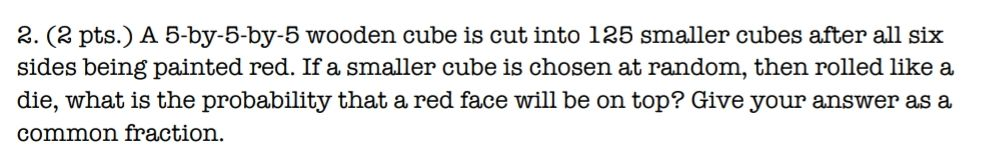### ¿Todavía tienes preguntas de matemáticas?

Pregunte a nuestros tutores expertos
Algebra
Pregunta2. (2 pts.) A 5-by-5-by-5 wooden cube is cut into $$125$$ smaller cubes after all six sides being painted red. If a smaller cube is chosen at random, then rolled like a die, what is the probability that a red face will be on top? Give your answer as a common fraction.

$$\frac{1}{6}$$